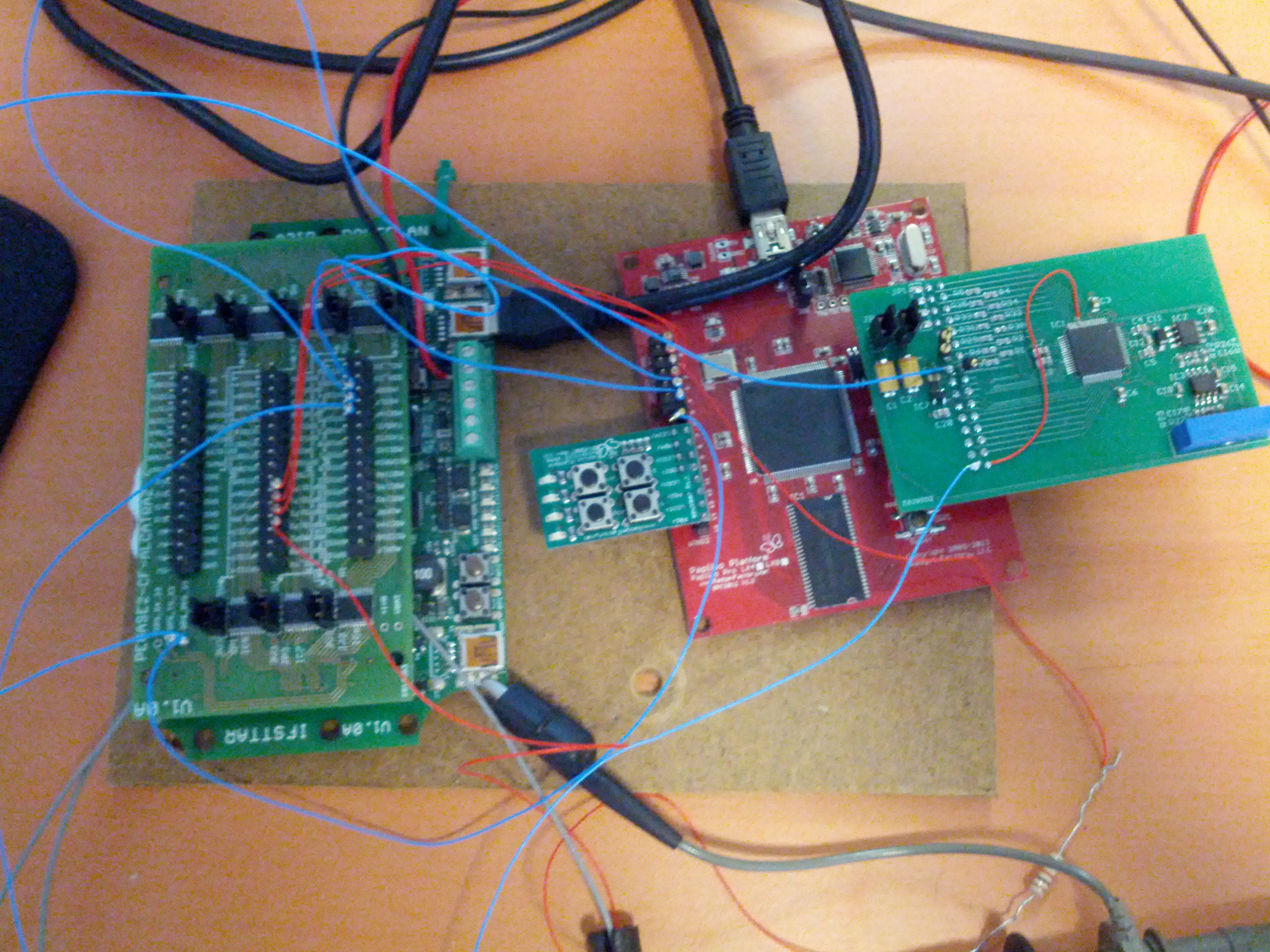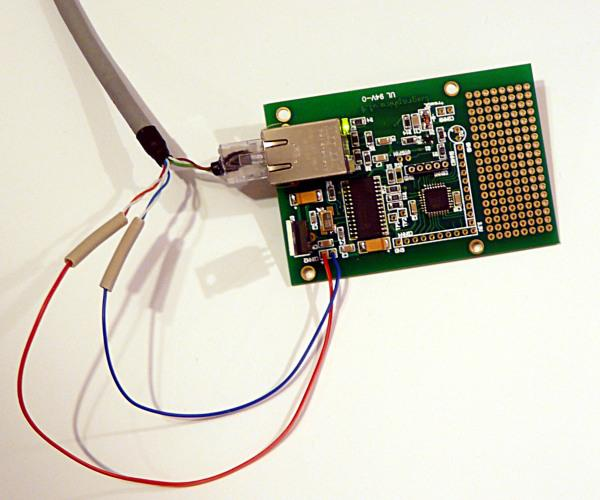##### I4S - 2014
Application Domains
New Software and Platforms
Bilateral Contracts and Grants with Industry
Partnerships and Cooperations
Bibliography
Application Domains
New Software and Platforms
Bilateral Contracts and Grants with Industry
Partnerships and Cooperations
Bibliography

## Section: Research Program

### Subspace-based identification and detection

For reasons closely related to the vibrations monitoring applications, we have been investigating subspace-based methods, for both the identification and the monitoring of the eigenstructure $\left(\lambda ,{\phi }_{\lambda }\right)$ of the state transition matrix $F$ of a linear dynamical state-space system :

 $\left\{\begin{array}{ccc}\hfill {X}_{k+1}& =& F\phantom{\rule{3.33333pt}{0ex}}{X}_{k}+{V}_{k+1}\hfill \\ \hfill {Y}_{k}& =& H\phantom{\rule{3.33333pt}{0ex}}{X}_{k}\hfill \end{array}\right\,$ (10)

namely the $\left(\lambda ,{\varphi }_{\lambda }\right)$ defined by :

 $det\phantom{\rule{3.33333pt}{0ex}}\left(F-\lambda \phantom{\rule{3.33333pt}{0ex}}I\right)=0,\phantom{\rule{3.33333pt}{0ex}}\phantom{\rule{3.33333pt}{0ex}}\left(F-\lambda \phantom{\rule{3.33333pt}{0ex}}I\right)\phantom{\rule{3.33333pt}{0ex}}{\phi }_{\lambda }=0,\phantom{\rule{3.33333pt}{0ex}}\phantom{\rule{3.33333pt}{0ex}}{\varphi }_{\lambda }\stackrel{\Delta }{=}H\phantom{\rule{3.33333pt}{0ex}}{\phi }_{\lambda }$ (11)

The (canonical) parameter vector in that case is :

 $\theta \stackrel{\Delta }{=}\left(\begin{array}{c}\Lambda \\ \mathrm{vec}\Phi \end{array}\right)$ (12)

where $\Lambda$ is the vector whose elements are the eigenvalues $\lambda$, $\Phi$ is the matrix whose columns are the ${\varphi }_{\lambda }$'s, and $\mathrm{vec}$ is the column stacking operator.

Subspace-based methods is the generic name for linear systems identification algorithms based on either time domain measurements or output covariance matrices, in which different subspaces of Gaussian random vectors play a key role   . A contribution of ours, minor but extremely fruitful, has been to write the output-only covariance-driven subspace identification method under a form that involves a parameter estimating function, from which we define a residual adapted to vibration monitoring  . This is explained next.

#### Covariance-driven subspace identification.

Let ${R}_{i}\stackrel{\Delta }{=}𝐄\left({Y}_{k}\phantom{\rule{3.33333pt}{0ex}}{Y}_{k-i}^{T}\right)$ and:

 ${ℋ}_{p+1,q}\stackrel{\Delta }{=}\left(\begin{array}{cccc}{R}_{0}& {R}_{1}& \phantom{\rule{-0.166667em}{0ex}}⋮\phantom{\rule{-0.166667em}{0ex}}& {R}_{q-1}\\ {R}_{1}& {R}_{2}& \phantom{\rule{-0.166667em}{0ex}}⋮\phantom{\rule{-0.166667em}{0ex}}& {R}_{q}\\ ⋮& ⋮& \phantom{\rule{-0.166667em}{0ex}}⋮\phantom{\rule{-0.166667em}{0ex}}& ⋮\\ {R}_{p}& {R}_{p+1}& \phantom{\rule{-0.166667em}{0ex}}⋮\phantom{\rule{-0.166667em}{0ex}}& {R}_{p+q-1}\end{array}\right)\stackrel{\Delta }{=}\mathrm{Hank}\left({R}_{i}\right)$ (13)

be the output covariance and Hankel matrices, respectively; and: $G\stackrel{\Delta }{=}𝐄\left({X}_{k}{Y}_{k}^{T}\right)$. Direct computations of the ${R}_{i}$'s from the equations (10 ) lead to the well known key factorizations :

 $\begin{array}{ccc}\hfill {R}_{i}& =& H{F}^{i}G\hfill \\ \hfill {ℋ}_{p+1,q}& =& {𝒪}_{p+1}\left(H,F\right)\phantom{\rule{3.33333pt}{0ex}}{𝒞}_{q}\left(F,G\right)\hfill \end{array}$ (14)

where:

 ${𝒪}_{p+1}\left(H,F\right)\stackrel{\Delta }{=}\left(\begin{array}{c}H\hfill \\ HF\hfill \\ \phantom{\rule{-0.166667em}{0ex}}⋮\phantom{\rule{-0.166667em}{0ex}}\hfill \\ H{F}^{p}\hfill \end{array}\right)\phantom{\rule{3.33333pt}{0ex}}\phantom{\rule{3.33333pt}{0ex}}\phantom{\rule{3.33333pt}{0ex}}\text{and}\phantom{\rule{3.33333pt}{0ex}}\phantom{\rule{3.33333pt}{0ex}}\phantom{\rule{3.33333pt}{0ex}}{𝒞}_{q}\left(F,G\right)\phantom{\rule{-0.166667em}{0ex}}\stackrel{\Delta }{=}\phantom{\rule{-0.166667em}{0ex}}\left(G\phantom{\rule{3.33333pt}{0ex}}FG\phantom{\rule{3.33333pt}{0ex}}\cdots \phantom{\rule{3.33333pt}{0ex}}{F}^{q-1}G\right)$ (15)

are the observability and controllability matrices, respectively. The observation matrix $H$ is then found in the first block-row of the observability matrix $𝒪$. The state-transition matrix $F$ is obtained from the shift invariance property of $𝒪$. The eigenstructure $\left(\lambda ,{\phi }_{\lambda }\right)$ then results from (11 ).

Since the actual model order is generally not known, this procedure is run with increasing model orders.

#### Model parameter characterization.

Choosing the eigenvectors of matrix $F$ as a basis for the state space of model (10 ) yields the following representation of the observability matrix:

 ${𝒪}_{p+1}\left(\theta \right)=\left(\begin{array}{c}\Phi \hfill \\ \Phi \Delta \hfill \\ ⋮\hfill \\ \Phi {\Delta }^{p}\hfill \end{array}\right)$ (16)

where $\Delta \stackrel{\Delta }{=}\mathrm{diag}\left(\Lambda \right)$, and $\Lambda$ and $\Phi$ are as in (12 ). Whether a nominal parameter ${\theta }_{0}$ fits a given output covariance sequence ${\left({R}_{j}\right)}_{j}$ is characterized by  :

 ${𝒪}_{p+1}\left({\theta }_{0}\right)\phantom{\rule{3.33333pt}{0ex}}\phantom{\rule{3.33333pt}{0ex}}\text{and}\phantom{\rule{3.33333pt}{0ex}}\phantom{\rule{3.33333pt}{0ex}}{ℋ}_{p+1,q}\phantom{\rule{3.33333pt}{0ex}}\phantom{\rule{3.33333pt}{0ex}}\text{have}\phantom{\rule{4.pt}{0ex}}\text{the}\phantom{\rule{4.pt}{0ex}}\text{same}\phantom{\rule{4.pt}{0ex}}\text{left}\phantom{\rule{4.pt}{0ex}}\text{kernel}\phantom{\rule{4.pt}{0ex}}\text{space.}$ (17)

This property can be checked as follows. From the nominal ${\theta }_{0}$, compute ${𝒪}_{p+1}\left({\theta }_{0}\right)$ using (16 ), and perform e.g. a singular value decomposition (SVD) of ${𝒪}_{p+1}\left({\theta }_{0}\right)$ for extracting a matrix $U$ such that:

 ${U}^{T}\phantom{\rule{0.277778em}{0ex}}U={I}_{s}\phantom{\rule{3.33333pt}{0ex}}\phantom{\rule{3.33333pt}{0ex}}\text{and}\phantom{\rule{3.33333pt}{0ex}}\phantom{\rule{3.33333pt}{0ex}}{U}^{T}\phantom{\rule{0.277778em}{0ex}}{𝒪}_{p+1}\left({\theta }_{0}\right)=0$ (18)

Matrix $U$ is not unique (two such matrices relate through a post-multiplication with an orthonormal matrix), but can be regarded as a function of ${\theta }_{0}$. Then the characterization writes:

 $U{\left({\theta }_{0}\right)}^{T}\phantom{\rule{0.277778em}{0ex}}{ℋ}_{p+1,q}=0$ (19)

#### Residual associated with subspace identification.

Assume now that a reference ${\theta }_{0}$ and a new sample ${Y}_{1},\cdots ,{Y}_{N}$ are available. For checking whether the data agree with ${\theta }_{0}$, the idea is to compute the empirical Hankel matrix ${\stackrel{^}{ℋ}}_{p+1,q}$:

 ${\stackrel{^}{ℋ}}_{p+1,q}\stackrel{\Delta }{=}\mathrm{Hank}\left({\stackrel{^}{R}}_{i}\right),\phantom{\rule{3.33333pt}{0ex}}\phantom{\rule{3.33333pt}{0ex}}\phantom{\rule{3.33333pt}{0ex}}{\stackrel{^}{R}}_{i}\stackrel{\Delta }{=}1/\left(N-i\right)\phantom{\rule{3.33333pt}{0ex}}\sum _{k=i+1}^{N}{Y}_{k}\phantom{\rule{3.33333pt}{0ex}}{Y}_{k-i}^{T}$ (20)

and to define the residual vector:

 ${\zeta }_{N}\left({\theta }_{0}\right)\stackrel{\Delta }{=}\sqrt{N}\phantom{\rule{3.33333pt}{0ex}}\mathrm{vec}\left(U{\left({\theta }_{0}\right)}^{T}\phantom{\rule{3.33333pt}{0ex}}{\stackrel{^}{ℋ}}_{p+1,q}\right)$ (21)

Let $\theta$ be the actual parameter value for the system which generated the new data sample, and ${𝐄}_{\theta }$ be the expectation when the actual system parameter is $\theta$. From (19 ), we know that ${\zeta }_{N}\left({\theta }_{0}\right)$ has zero mean when no change occurs in $\theta$, and nonzero mean if a change occurs. Thus ${\zeta }_{N}\left({\theta }_{0}\right)$ plays the role of a residual.

It is our experience that this residual has highly interesting properties, both for damage detection  and localization  , and for flutter monitoring  .

#### Other uses of the key factorizations.

Factorization ( 3.5.1 ) is the key for a characterization of the canonical parameter vector $\theta$ in (12 ), and for deriving the residual. Factorization (14 ) is also the key for :

• Proving consistency and robustness results  ;

• Designing an extension of covariance-driven subspace identification algorithm adapted to the presence and fusion of non-simultaneously recorded multiple sensors setups  ;

• Proving the consistency and robustness of this extension  ;

• Designing various forms of input-output covariance-driven subspace identification algorithms adapted to the presence of both known inputs and unknown excitations  .

#### Research program

The research will first focus on the extension and implementation of current techniques as developed in I4S and IFSTTAR. Before doing any temperature rejection on large scale structures as planned, we need to develop good and accurate models of thermal fields. We also need to develop robust and efficient versions of our algorithms, mainly the subspace algorithms before envisioning linking them with physical models. Briefly, we need to mature our statistical toolset as well as our physical modeling before mixing them together later on.

##### Direct vibration modeling under temperature changes

This task builds upon what has been achieved in the CONSTRUCTIF project, where a simple formulation of the temperature effect has been exhibited, based on relatively simple assumptions. The next step is to generalize this modeling to a realistic large structure under complex thermal changes. Practically, temperature and resulting structural prestress and pre strains of thermal origin are not uniform and civil structures are complex. This leads to a fully 3D temperature field, not just a single value. Inertia effects also forbid a trivial prediction of the temperature based on current sensor outputs while ignoring past data. On the other side, the temperature is seen as a nuisance. That implies that any damage detection procedure has first to correct the temperature effect prior to any detection.

Modeling vibrations of structures under thermal prestress does and will play an important role in the static correction of kinematic measurements, in health monitoring methods based on vibration analysis as well as in durability and in the active or semi-active control of civil structures that by nature are operated under changing environmental conditions. As a matter of fact, using temperature and dynamic models the project aims at correcting the current vibration state from induced temperature effects, such that damage detection algorithms rely on a comparison of this thermally corrected current vibration state with a reference state computed or measured at a reference temperature. This approach is expected to cure damage detection algorithms from the environmental variations.

I4S will explore various ways of implementing this concept, notably within the FUI SIPRIS project.

##### Damage localization algorithms (in the case of localized damages such as cracks)

During the CONSTRUCTIF project, both feasibility and efficiency of some damage detection and localization algorithms were proved. Those methods are based on the tight coupling of statistical algorithms with finite element models. It has been shown that effective localization of some damaged elements was possible, and this was validated on a numerical simulated bridge deck model. Still, this approach has to be validated on real structures.

On the other side, new localization algorithms are currently investigated such as the one developed conjointly with University of Boston and tested within the framework of FP7 ISMS project. These algorithms will be implemented and tested on the PEGASE platform as well as all our toolset.

When possible, link with temperature rejection will be done along the lines of what has been achieved in the CONSTRUCTIF project.

##### Uncertainty quantification for system identification algorithms

Some emphasis will be put on expressing confidence intervals for system identification. It is a primary goal to take into account the uncertainty within the identification procedure, using either identification algorithms derivations or damage detection principles. Such algorithms are critical for both civil and aeronautical structures monitoring. It has been shown that confidence intervals for estimation parameters can theoretically be related to the damage detection techniques and should be computed as a function of the Fisher information matrix associated to the damage detection test. Based on those assumptions, it should be possible to obtain confidence intervals for a large class of estimates, from damping to finite elements models. Uncertainty considerations are also deeply investigated in collaboration with Dassault Aviation in Mellinger PhD thesis or with Northeastern University, Boston, within Gallegos PhD thesis.

##### Reflectometry-based methods for civil engineering structure health monitoring

For mechanical structures with a dominating geometrical axis so that they can be approximately considered one dimensional structures, some reflectometry-based methods initially developed for electrical cable monitoring have proved efficient for their health monitoring. Typical applications of such methods have been validated for the monitoring of external post-tensioned cables built with concrete bridges. Further studies are necessary to generalize this technology to other mechanical structures.

##### PEGASE platform

A new iteration called PEGASE 2 of our wireless platform has to be finalized (see Software section), in particular:

• Validation of PEGASE 2 mother board for its ability to recover energy from solar cells. Writing resulting abacus and user-guides...

• Discover and manage the DSP Library of PEGASE 2 (TI 5330 processor)

• Finalizing its main daughter boards:

• 8 synchronous analog channel daughter board (finalized at 90

• validation of the POE (Power Over Ethernet) daughter board

• validation of the 3G daughter board (for GSM links)

• Finalizing the supervisor (Matlab plugin...)

Figure 1. PEGASE board